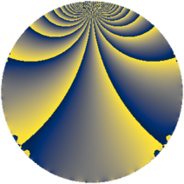# Properties

 Label 608.1.oLevel $608$ Weight $1$ Character orbit 608.o Rep. character $\chi_{608}(239,\cdot)$ Character field $\Q(\zeta_{6})$ Dimension $2$ Newform subspaces $1$ Sturm bound $80$ Trace bound $0$

# Related objects

## Defining parameters

 Level: $$N$$ $$=$$ $$608 = 2^{5} \cdot 19$$ Weight: $$k$$ $$=$$ $$1$$ Character orbit: $$[\chi]$$ $$=$$ 608.o (of order $$6$$ and degree $$2$$) Character conductor: $$\operatorname{cond}(\chi)$$ $$=$$ $$152$$ Character field: $$\Q(\zeta_{6})$$ Newform subspaces: $$1$$ Sturm bound: $$80$$ Trace bound: $$0$$

## Dimensions

The following table gives the dimensions of various subspaces of $$M_{1}(608, [\chi])$$.

Total New Old
Modular forms 24 6 18
Cusp forms 8 2 6
Eisenstein series 16 4 12

The following table gives the dimensions of subspaces with specified projective image type.

$$D_n$$ $$A_4$$ $$S_4$$ $$A_5$$
Dimension 2 0 0 0

## Trace form

 $$2 q - q^{3} + O(q^{10})$$ $$2 q - q^{3} + 2 q^{11} - 2 q^{17} + q^{19} - q^{25} - 2 q^{27} - q^{33} + q^{41} + 2 q^{43} + 2 q^{49} - 2 q^{51} - 2 q^{57} - q^{59} - q^{67} + q^{73} + 2 q^{75} + q^{81} + 2 q^{83} - 2 q^{89} + q^{97} + O(q^{100})$$

## Decomposition of $$S_{1}^{\mathrm{new}}(608, [\chi])$$ into newform subspaces

Label Dim $A$ Field Image CM RM Traces $q$-expansion
$a_{2}$ $a_{3}$ $a_{5}$ $a_{7}$
608.1.o.a $2$ $0.303$ $$\Q(\sqrt{-3})$$ $D_{3}$ $$\Q(\sqrt{-2})$$ None $$0$$ $$-1$$ $$0$$ $$0$$ $$q+\zeta_{6}^{2}q^{3}+q^{11}+\zeta_{6}^{2}q^{17}+\zeta_{6}q^{19}+\cdots$$

## Decomposition of $$S_{1}^{\mathrm{old}}(608, [\chi])$$ into lower level spaces

$$S_{1}^{\mathrm{old}}(608, [\chi]) \cong$$ $$S_{1}^{\mathrm{new}}(152, [\chi])$$$$^{\oplus 3}$$